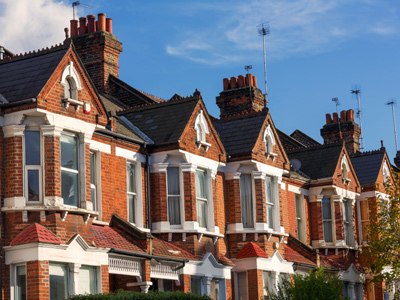If 3 out of 10 people live in a terraced house, what percentage does this represent?

# Percentages (Difficult)

Percentages are proportions of numbers. In this second section of quizzes on percentages, you'll get some practice in expressing one number as a percentage of another number. Suppose you were asked: 'What percentage of 50 is 20?' How would you do it? This is how you would do it: 2050 × 100% = (20 × 100) ÷ 50 = 40%.

Percentages are an important part of Maths and they crop up in everyday life all the time. You may have heard a relative talk about a 5 or 10% deposit on a house, or perhaps interest on a bank loan. It's a good idea to really learn about percentages now, because as you progress through school you'll be asked to do harder and harder calculations involving percentages. They are all around us - and they won't go away!

See if you can get 100% in this 11-plus Maths quiz! As always, take your time, read the question and four answers carefully before making your choice.

1.
If, in a certain survey, 25 out of 125 people like Crunchy Crunch chocolate, what percentage of those surveyed does this represent?
3.125%
20%
2%
31.25%
25125 × 100% = (25 × 100) ÷ 125 = 20%
2.
If 240 people out of a workforce of 1,500 were fired, what percentage of the workforce does this represent?
16%
36%
1.6%
3.6%
2401,500 × 100% = (240 × 100) ÷ 1,500 = 16%
3.
If, in a survey on spending habits, 200 out of 10,000 people said they spent more than £300 a week on entertainment, what percentage does this represent?
20%
2%
200%
0.2%
20010,000 × 100% = (200 × 100) ÷ 10,000 = 2%
4.
If 12 pupils in a class of 30 don't have school lunch, what percentage of the class does this represent?
40%
36%
3.6%
4%
1230 × 100% = (12 × 100) ÷ 30 = 40%
5.
What percentage of 48 is 15?
72%
7.2%
3.125%
31.25%
1548 × 100% = (15 × 100) ÷ 48 = 31.25%
6.
What percentage of 500 is 60?
3%
30%
12%
1.2%
60500 × 100% = (60 × 100) ÷ 500 = 12%
7.
What percentage of 300 is 900?
30%
270%
300%
27%
90030 × 100% = (900 × 100) ÷ 30 = 300%. DON'T think that percentages can't be more than 100%
8.
If 3 out of 10 people live in a terraced house, what percentage does this represent?
0.3%
3%
300%
30%
310 × 100% = (3 × 100) ÷ 10 = 30%
9.
What percentage of 90 is 18?
1.62%
20%
2%
16.2%
1890 × 100% = (18 × 100) ÷ 90 = 20%
10.
What percentage of 100 is 20?
0.2%
20%
200%
10%
20100 × 100% = (20 × 100) ÷ 100 = 20%
Author:  Frank Evans